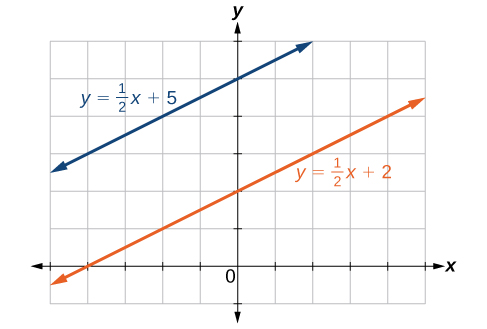# 2.2 Linear equations in one variable  (Page 6/15)

 Page 6 / 15

Find the equation of the line passing through $\text{\hspace{0.17em}}\left(-5,2\right)\text{\hspace{0.17em}}$ and $\text{\hspace{0.17em}}\left(2,2\right).$

Horizontal line: $\text{\hspace{0.17em}}y=2$

## Determining whether graphs of lines are parallel or perpendicular

Parallel lines have the same slope and different y- intercepts. Lines that are parallel to each other will never intersect. For example, [link] shows the graphs of various lines with the same slope, $\text{\hspace{0.17em}}m=2.$

All of the lines shown in the graph are parallel because they have the same slope and different y- intercepts.

Lines that are perpendicular intersect to form a $\text{\hspace{0.17em}}90°$ -angle. The slope of one line is the negative reciprocal of the other. We can show that two lines are perpendicular if the product of the two slopes is $\text{\hspace{0.17em}}-1:{m}_{1}\cdot {m}_{2}=-1.\text{\hspace{0.17em}}$ For example, [link] shows the graph of two perpendicular lines. One line has a slope of 3; the other line has a slope of $\text{\hspace{0.17em}}-\frac{1}{3}.$

$\begin{array}{ccc}\hfill {m}_{1}\cdot {m}_{2}& =& -1\hfill \\ \hfill 3\cdot \left(-\frac{1}{3}\right)& =& -1\hfill \end{array}$

## Graphing two equations, and determining whether the lines are parallel, perpendicular, or neither

Graph the equations of the given lines, and state whether they are parallel, perpendicular, or neither: $\text{\hspace{0.17em}}3y=-4x+3\text{\hspace{0.17em}}$ and $\text{\hspace{0.17em}}3x-4y=8.$

The first thing we want to do is rewrite the equations so that both equations are in slope-intercept form.

First equation:

$\begin{array}{ccc}\hfill 3y& =& -4x+3\hfill \\ \hfill y& =& -\frac{4}{3}x+1\hfill \end{array}$

Second equation:

$\begin{array}{ccc}\hfill 3x-4y& =& 8\hfill \\ \hfill -4y& =& -3x+8\hfill \\ \hfill y& =& \frac{3}{4}x-2\hfill \end{array}$

See the graph of both lines in [link]

From the graph, we can see that the lines appear perpendicular, but we must compare the slopes.

$\begin{array}{ccc}\hfill {m}_{1}& =& -\frac{4}{3}\hfill \\ \hfill {m}_{2}& =& \frac{3}{4}\hfill \\ \hfill {m}_{1}\cdot {m}_{2}& =& \left(-\frac{4}{3}\right)\left(\frac{3}{4}\right)=-1\hfill \end{array}$

The slopes are negative reciprocals of each other, confirming that the lines are perpendicular.

Graph the two lines and determine whether they are parallel, perpendicular, or neither: $\text{\hspace{0.17em}}2y-x=10\text{\hspace{0.17em}}$ and $\text{\hspace{0.17em}}2y=x+4.$

Parallel lines: equations are written in slope-intercept form.## Writing the equations of lines parallel or perpendicular to a given line

As we have learned, determining whether two lines are parallel or perpendicular is a matter of finding the slopes. To write the equation of a line parallel or perpendicular to another line, we follow the same principles as we do for finding the equation of any line. After finding the slope, use the point-slope formula to write the equation of the new line.

Given an equation for a line, write the equation of a line parallel or perpendicular to it.

1. Find the slope of the given line. The easiest way to do this is to write the equation in slope-intercept form.
2. Use the slope and the given point with the point-slope formula.
3. Simplify the line to slope-intercept form and compare the equation to the given line.

## Writing the equation of a line parallel to a given line passing through a given point

Write the equation of line parallel to a $\text{\hspace{0.17em}}5x+3y=1\text{\hspace{0.17em}}$ and passing through the point $\text{\hspace{0.17em}}\left(3,5\right).$

First, we will write the equation in slope-intercept form to find the slope.

$\begin{array}{ccc}\hfill 5x+3y& =& 1\hfill \\ \hfill 3y& =& 5x+1\hfill \\ \hfill y& =& -\frac{5}{3}x+\frac{1}{3}\hfill \end{array}$

The slope is $\text{\hspace{0.17em}}m=-\frac{5}{3}.\text{\hspace{0.17em}}$ The y- intercept is $\text{\hspace{0.17em}}\frac{1}{3},$ but that really does not enter into our problem, as the only thing we need for two lines to be parallel is the same slope. The one exception is that if the y- intercepts are the same, then the two lines are the same line. The next step is to use this slope and the given point with the point-slope formula.

$\begin{array}{ccc}\hfill y-5& =& -\frac{5}{3}\left(x-3\right)\hfill \\ \hfill y-5& =& -\frac{5}{3}x+5\hfill \\ \hfill y& =& -\frac{5}{3}x+10\hfill \end{array}$

The equation of the line is $\text{\hspace{0.17em}}y=-\frac{5}{3}x+10.\text{\hspace{0.17em}}$ See [link] .

#### Questions & Answers

write down the polynomial function with root 1/3,2,-3 with solution
if A and B are subspaces of V prove that (A+B)/B=A/(A-B)
write down the value of each of the following in surd form a)cos(-65°) b)sin(-180°)c)tan(225°)d)tan(135°)
Prove that (sinA/1-cosA - 1-cosA/sinA) (cosA/1-sinA - 1-sinA/cosA) = 4
what is the answer to dividing negative index
In a triangle ABC prove that. (b+c)cosA+(c+a)cosB+(a+b)cisC=a+b+c.
give me the waec 2019 questions
the polar co-ordinate of the point (-1, -1)
prove the identites sin x ( 1+ tan x )+ cos x ( 1+ cot x )= sec x + cosec x
tanh`(x-iy) =A+iB, find A and B
B=Ai-itan(hx-hiy)
Rukmini
what is the addition of 101011 with 101010
If those numbers are binary, it's 1010101. If they are base 10, it's 202021.
Jack
extra power 4 minus 5 x cube + 7 x square minus 5 x + 1 equal to zero
the gradient function of a curve is 2x+4 and the curve passes through point (1,4) find the equation of the curve
1+cos²A/cos²A=2cosec²A-1
test for convergence the series 1+x/2+2!/9x3ByByBy DanielrosenbergerBy